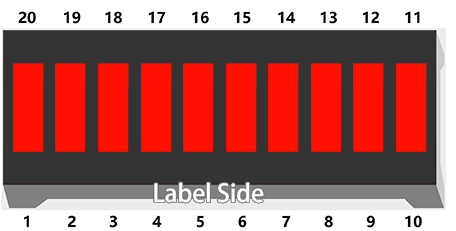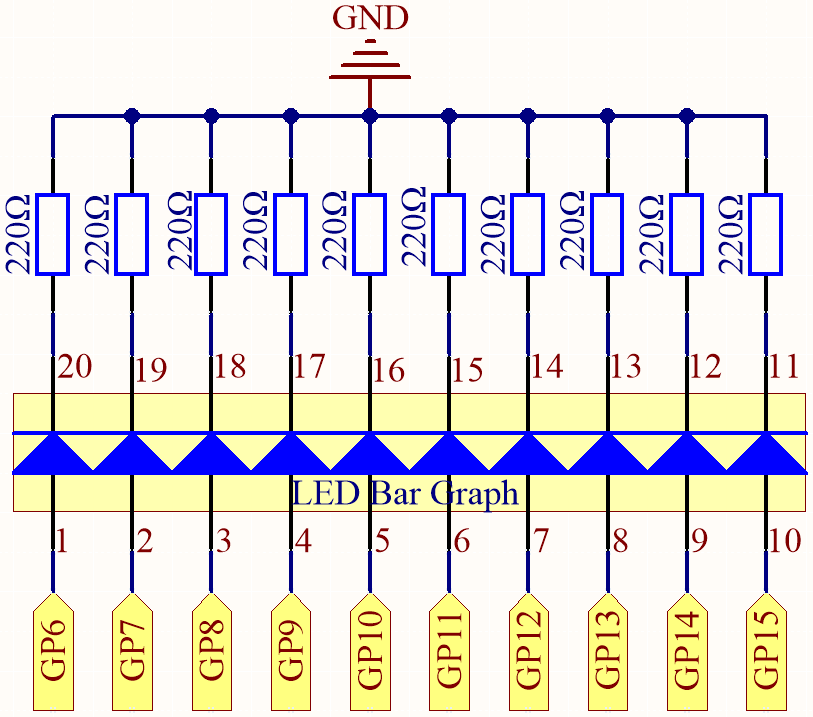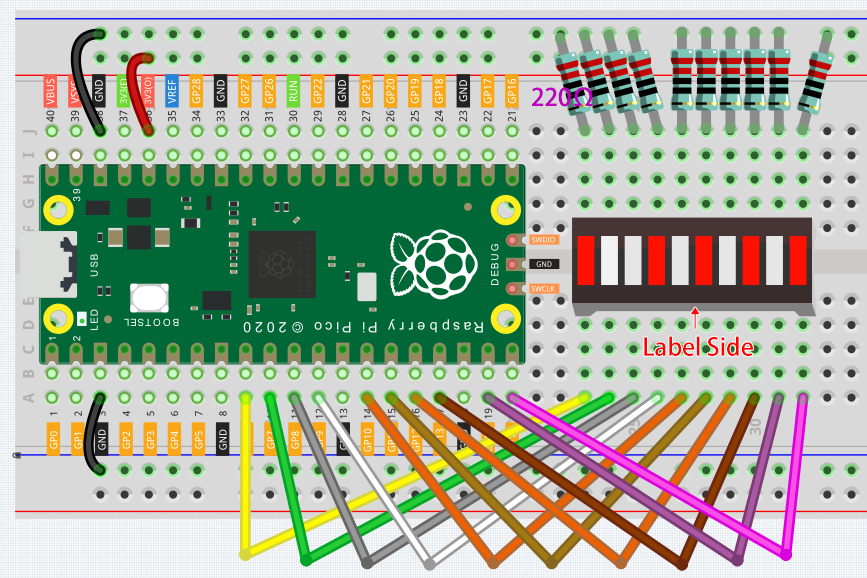# 2.2 - Display the Level¶

The first project is simply to make the LED blink. In this project let’s use the LED Bar Graph, which is made up of 10 LEDs packaged into a plastic case, generally used to display power or volume levels.SchematicThe LED Bar Graph contains 10 LEDs, each of which is individually controllable. Here, the anode of each of the 10 LEDs is connected to GP6~GP15, and the cathode is connected to a 220ohm resistor, and then to GND.

WiringCode

Note

• You can open the file `2.2_display_the_level.ino` under the path of `euler-kit/arduino/2.2_display_the_level`.

• Or copy this code into Arduino IDE.

• For detailed tutorials, please refer to Open & Run Code Directly.

• Or run this code directly in the Arduino Web Editor.

Don’t forget to select the Raspberry Pi Pico board and the correct port before clicking the Upload button.

When the program is running, you will see the LEDs on the LED Bar Graph light up and then turn off in sequence.

How it works?

Each of the ten LEDs on the LED Bar needs to be controlled by a pin, which means that we define these ten pins.

The codes in `setup()` use the for loop to initialize pins 6~15 to output mode in turn.

```for(int i=6;i<=15;i++)
{
pinMode(i,OUTPUT);
}
```

The for loop is used in `loop()` to make the LED flash(turn on 0.5s, then turn off 0.5s) in sequence.

```for(int i=6;i<=15;i++)
{
digitalWrite(i,HIGH);
delay(500);
digitalWrite(i,LOW);
delay(500);
}
```